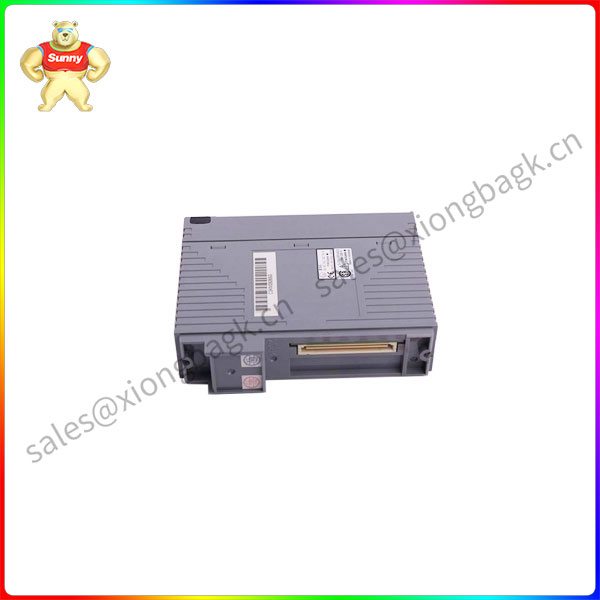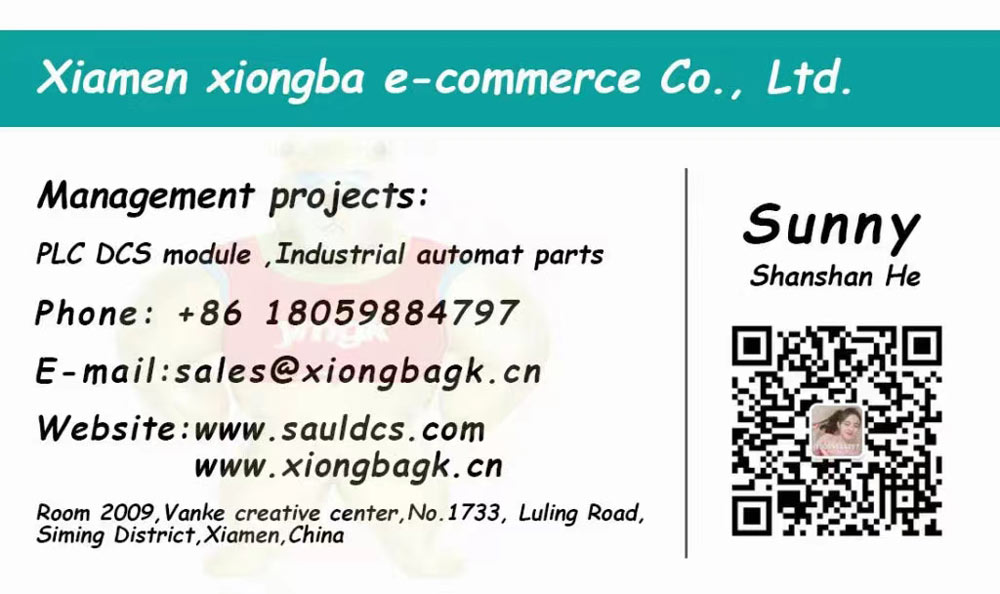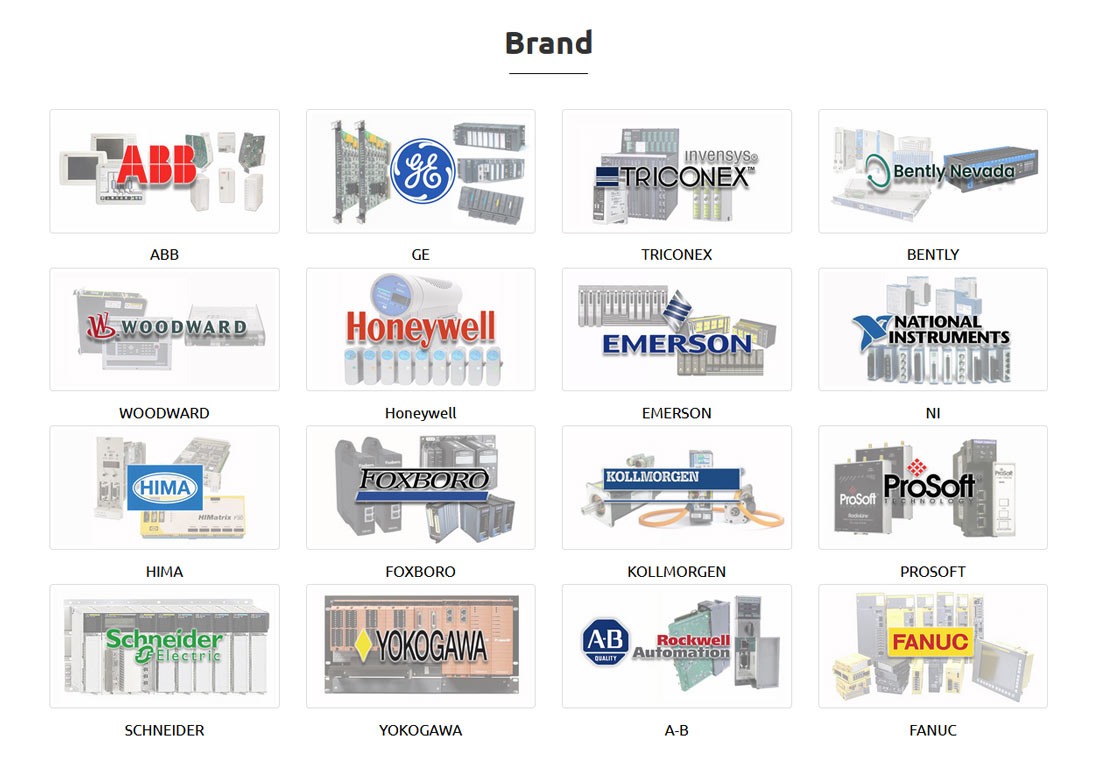# PW702 YOKOGAWA Dc power module

PW702 Dc power supply is a device that can maintain a stable voltage and current in the circuit, which can convert AC

power supply into DC power supply and provide stable DC power supply for various electronic devices. Common

DC power supplies include dry batteries, storage batteries, DC generators, etc.

Category:

## PW702 YOKOGAWA Dc power module

PW702 Dc power supply is a device that can maintain a stable voltage and current in the circuit, which can convert AC

power supply into DC power supply and provide stable DC power supply for various electronic devices. Common

DC power supplies include dry batteries, storage batteries, DC generators, etc.

PW702 The working principle of DC power supply is to achieve stable current output through electrostatic and non-electrostatic

interaction. Specifically, the charge distribution and interaction inside the DC power supply causes the positive charge

to return from the interior of the cathode with a low potential to the interior of the high potential to maintain the potential

difference between the two electrodes and form a stable current.

PW702 When the DC power supply is connected to the external circuit, the current from the positive electrode to the negative

electrode is formed due to the push of the external electric field force of the power supply (external circuit). Inside the

power supply (the internal circuit), the action of non-electrostatic forces causes the current to flow from the negative

electrode to the positive electrode, so that the charge flow forms a closed cycle.PW702

PW702 In order to obtain higher DC voltage, DC power supplies often use series mode, the total electromotive force is the

sum of the electromotive force of each power supply, and the total internal resistance is the sum of the resistance of

each power supply. Due to the increase in internal resistance, this DC power supply can only be used in circuits where

the required current strength is small. In order to obtain greater current strength, the equivalent electromotive force of the

PW702 DC power supply can be used in parallel, the total electromotive force is the electromotive force of a single power supply,

and the total internal resistance is the parallel value of each power supply resistance.

Different types of DC power supply have different non-electrostatic properties, and the process of energy conversion is

also different. For example, in a chemical battery, the electrostatic force is related to the process of dissolution and

deposition of chemical ions, and when the chemical battery is discharged, the chemical energy is converted into electrical

energy and joule heat to the power of the temperature difference. In a DC generator, the non-electrostatic force is the effect of electromagnetic induction.